# Using the method of successive subtraction examine whether or not the following numbers are perfect cubes:`
Question:

Using the method of successive subtraction examine whether or not the following numbers are perfect cubes:

(i) 130

(ii) 345

(iii) 792

(iv) 1331

Solution:

(i)
We have: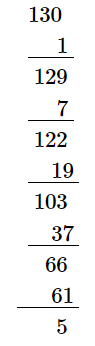$\because$ The next number to be subtracted is 91 , which is greater than 5 .

$\therefore 130$ is not a perfect cube.

(ii)
We have: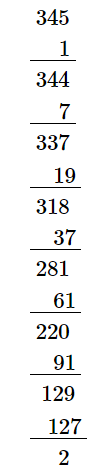$\because$ The next number to be subtracted is 161 , which is greater than 2 .

$\therefore 345$ is not a perfect cube.

(iii)
We have: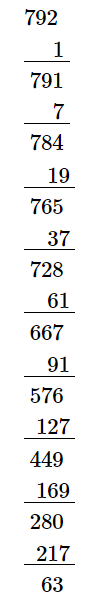$\because$ The next number to be subtracted is 271 , which is greater than 63 .

$\therefore 792$ is not a perfect cube.

(iv)
We have: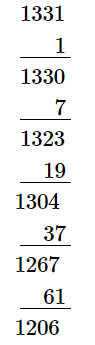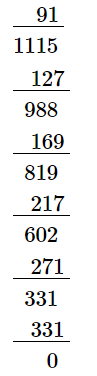$\because$ The subtraction is performed 11 times.

$\therefore \sqrt{1331}=11$

Thus, 1331 is a perfect cube.